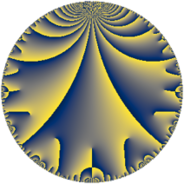# Properties

 Label 9.7.bLevel $9$ Weight $7$ Character orbit 9.b Rep. character $\chi_{9}(8,\cdot)$ Character field $\Q$ Dimension $2$ Newform subspaces $1$ Sturm bound $7$ Trace bound $0$

# Related objects

## Defining parameters

 Level: $$N$$ $$=$$ $$9 = 3^{2}$$ Weight: $$k$$ $$=$$ $$7$$ Character orbit: $$[\chi]$$ $$=$$ 9.b (of order $$2$$ and degree $$1$$) Character conductor: $$\operatorname{cond}(\chi)$$ $$=$$ $$3$$ Character field: $$\Q$$ Newform subspaces: $$1$$ Sturm bound: $$7$$ Trace bound: $$0$$

## Dimensions

The following table gives the dimensions of various subspaces of $$M_{7}(9, [\chi])$$.

Total New Old
Modular forms 8 2 6
Cusp forms 4 2 2
Eisenstein series 4 0 4

## Trace form

 $$2 q - 196 q^{4} + 1048 q^{7} + O(q^{10})$$ $$2 q - 196 q^{4} + 1048 q^{7} - 1620 q^{10} + 688 q^{13} - 1528 q^{16} - 4640 q^{19} + 22032 q^{22} + 23150 q^{25} - 102704 q^{28} - 21128 q^{31} + 181764 q^{34} - 48164 q^{37} + 55080 q^{40} - 181904 q^{43} - 146448 q^{46} + 313854 q^{49} - 67424 q^{52} + 110160 q^{55} - 589356 q^{58} + 502276 q^{61} + 854768 q^{64} - 432176 q^{67} - 848880 q^{70} - 616352 q^{73} + 454720 q^{76} - 1080248 q^{79} + 2770524 q^{82} + 908820 q^{85} - 749088 q^{88} + 360512 q^{91} - 3282768 q^{94} - 74336 q^{97} + O(q^{100})$$

## Decomposition of $$S_{7}^{\mathrm{new}}(9, [\chi])$$ into newform subspaces

Label Dim $A$ Field CM Traces $q$-expansion
$a_{2}$ $a_{3}$ $a_{5}$ $a_{7}$
9.7.b.a $2$ $2.070$ $$\Q(\sqrt{-2})$$ None $$0$$ $$0$$ $$0$$ $$1048$$ $$q+\beta q^{2}-98q^{4}+5\beta q^{5}+524q^{7}+\cdots$$

## Decomposition of $$S_{7}^{\mathrm{old}}(9, [\chi])$$ into lower level spaces

$$S_{7}^{\mathrm{old}}(9, [\chi]) \cong$$ $$S_{7}^{\mathrm{new}}(3, [\chi])$$$$^{\oplus 2}$$